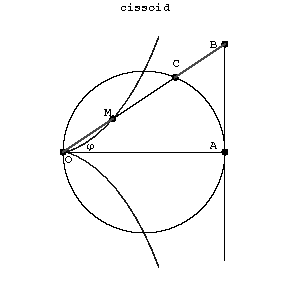# Cissoid

A plane algebraic curve of order three whose equation in Cartesian coordinates is

$$y^2=\frac{x^3}{2a-x}.$$

The parametric equations are

$$x=\frac{2at^2}{t^2+1},\qquad y=\frac{2at^3}{t^2+1}.$$

A cissoid is symmetric relative to the $x$-axis (Fig.). The coordinate origin is a cusp, the asymptote is $x=2a$. The area between the curve and the asymptote is $S=3\pi a^2$.Figure: c022340a

The cissoid is often called the cissoid of Diocles in honour of the Ancient Greek mathematician Diocles (3rd century B.C.), who discussed it in connection with the problem of duplication of the cube.

The cissoid is the set of points $M$ for which $OM=CB$, where $B$ and $C$ are the points of intersection of the line $OM$ with a circle and the tangent $AB$ to the circle at the point $A$ diametrically opposite to $O$. If in this construction one replaces the circle and straight line by curves $\rho_1=f_1(\phi)$ and $\rho_2=f_2(\phi)$, then the resulting curve $\rho=\rho_1-\rho_2$ is called a cissoidal curve, or the cissoid of the (given) curves.

How to Cite This Entry:
Cissoid. Encyclopedia of Mathematics. URL: http://encyclopediaofmath.org/index.php?title=Cissoid&oldid=43617
This article was adapted from an original article by D.D. Sokolov (originator), which appeared in Encyclopedia of Mathematics - ISBN 1402006098. See original article